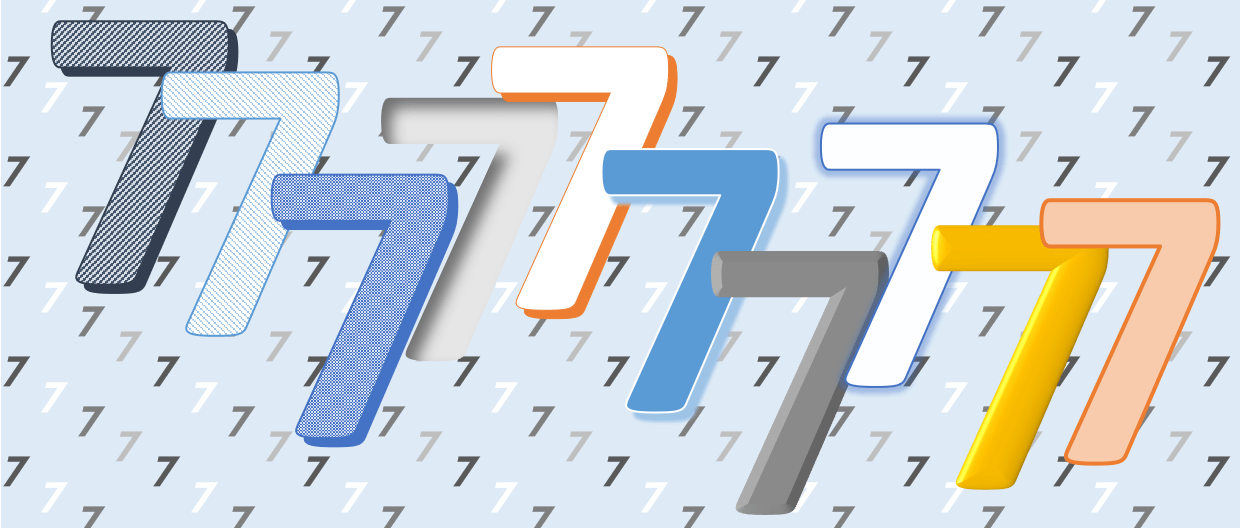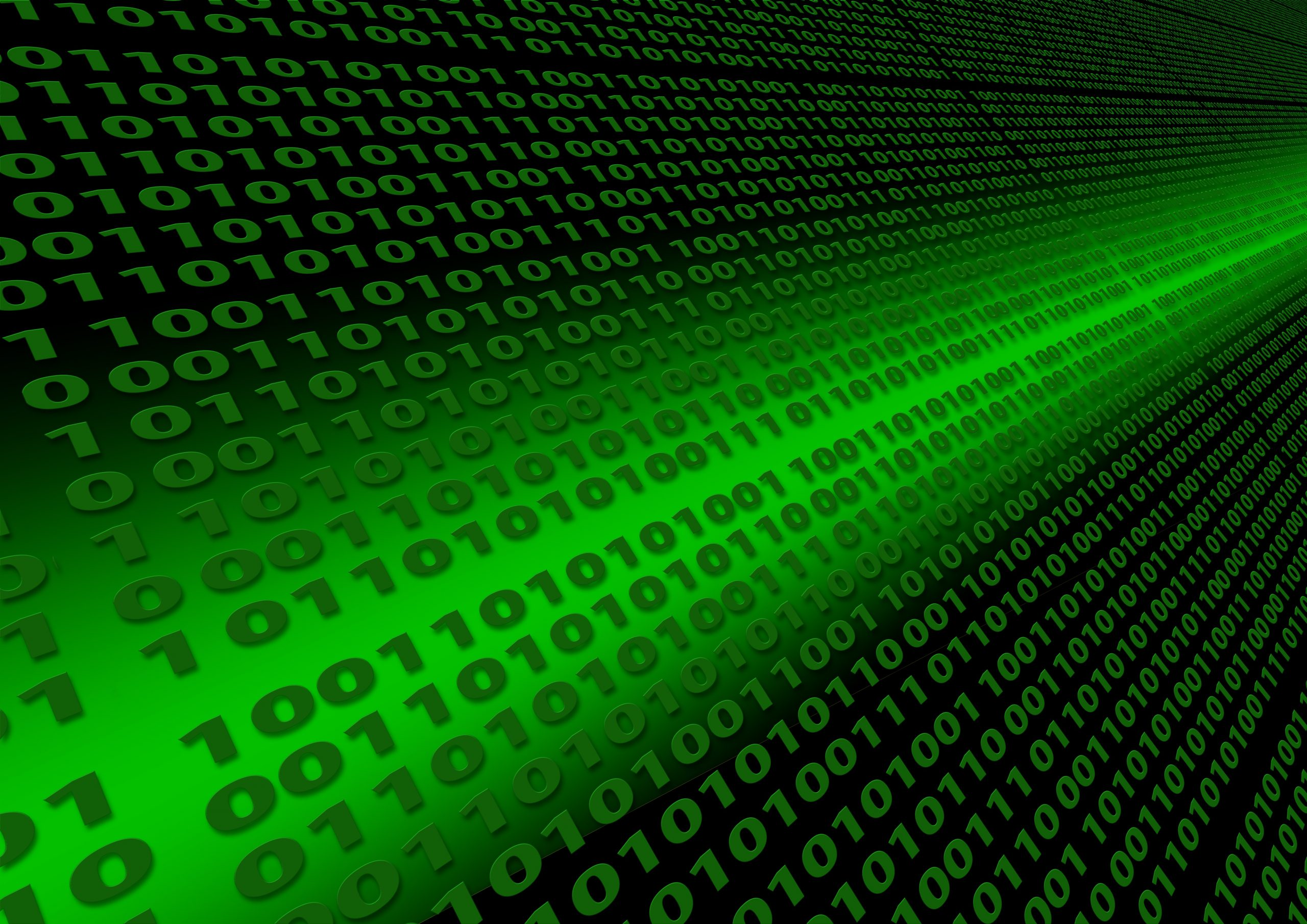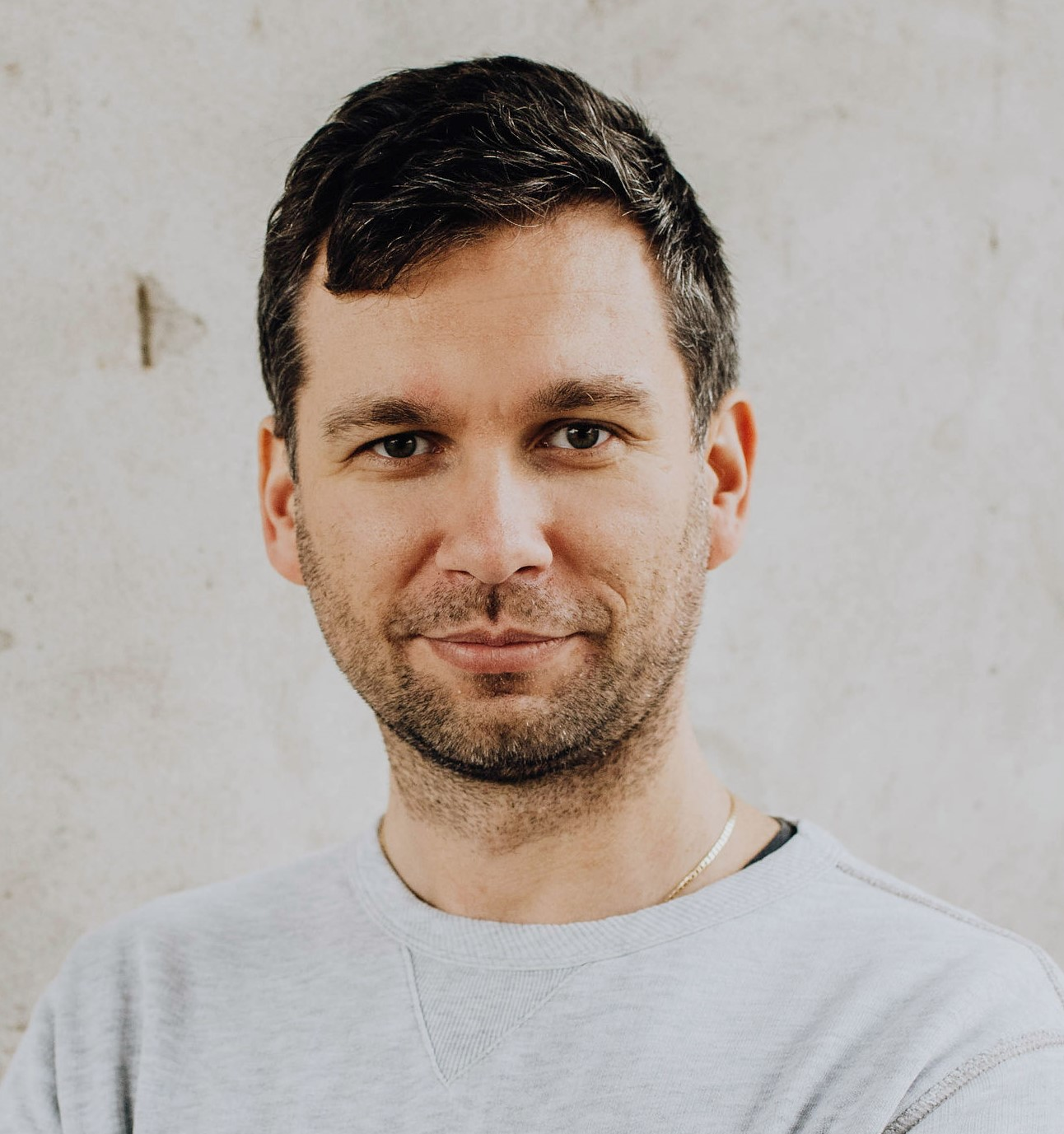# 7 ways to tell if a number is divisible by 7

Łukasz takes us on a tour through a surprisingly diverse range of algorithms to test for divisibility by 7I’m sure you know how to check if a huge number is divisible by 3, 9, 11 or by powers of 2 like 2, 4, and 8; you were probably taught how to do this in primary school. However most of you were probably never taught how to test whether a number is divisible by 7. In this article we will explore seven different ways to do that.7 is by far the least-loved single digit number when it comes to divisibility. Image: Chalkdust

Before we start, let’s introduce some notation which we’ll use throughout the article. An $n$-digit number $N$ in decimal base 10 is a number with digits belonging to the set $C_{10}=\{0, 1, 2, 3, 4, 5, 6, 7, 8, 9\}$ and can be expressed as sum of powers of 10: $N=\sum_{i=0}^{n-1}10^ic_i,$ where  $c_i \in C_{10}$. We normally write this number by concatenating the digits using their position to indicate the corresponding power of 10: $c_{n-1}c_{n-2}\cdots c_1c_0$. The least significant digit of $N$ is $c_0$, and most significant digit is $c_{n-1}$.

If $p$ is a positive integer we say the integers $a$ and $b$ are congruent modulo $p$ and write $a\equiv b\;(\textrm{mod}\; p)$ or $a\equiv_p b$ if they have the same remainder on division by $p$. For $2m$ integers $a_0,a_1,\dots,a_{m-1}$, $b_0,b_1,\dots,b_{m-1}$, we define:
$(a_0,a_1,\dots,a_{m-1})\equiv_p(b_0,b_1,\dots,b_{m-1})$ if and only if $a_i\equiv_p b_i\;\textrm{for}\; 0\le i\le m-1.$
We will also define two sums $S_1$ and $S_{-1}$ based on the digits of our number $N$:
$S_1=\sum_{i=0}^{n-1}c_i,\quad S_{-1}=\sum_{i=0}^{n-1}(-1)^ic_i.$
Note that the mechanisms behind the well-known divisibility tests for 3, 9, 11, and powers of two can be concisely expressed in terms of these sums as follows: $N\equiv_3 S_1$, $N\equiv_9 S_1$, $N\equiv_{11} S_{-1}$, and $N\equiv_{2^k} c_{k-1}\cdots c_1c_0$.

## Trimming method

The author of this technique is mathematician Antoni Żbikowski. In 1860 (Bulletin de l’Académie impériale des sciences de Saint Pétersbourg) and in 1861 (article Ułatwione sposoby rozpoznawania podzielności liczb (Facilitated ways to recognize divisibility of numbers), Polytechnic diary, Warsaw), he published an elementary method for determining when any given integer is divisible by any other $d$ which is coprime to 10.

For example if $N$ is expressed in form $N=10a+b$, then $N$ is divisible by 7 if and only if $a-2b$ is divisible by 7. The proof follows from the fact that $N$ is divisible by 7 if and only if $N-21b$ is divisible by 7.

If $N$ is at least a 3-digit number then replace $N$ by value of $\left\lfloor\frac{N}{10}\right\rfloor-2\times(N\;\textrm{mod}\;10)$. After each step, the number of digits is smaller than in previous step. Therefore, the number of steps is finite. When $N$ is no more than 2 digits in length, you can check divisibility by 7 straightforwardly if you know your 7 times table up to 14. Careful implementation of this approach costs linear time, and space complexity is linear too (see below). In general this technique doesn’t give the remainder when $N$ is divided by $d.$In general when designing an algorithm which you want to implement in practice, understanding its time and space complexity is vital. Image: public domain

In computer science, the time complexity measures the amount of time it takes to run an algorithm. An algorithm is said to take linear time, $O(n)$ time, if the running time increases at most linearly with the size of the input, ie the number of digits $n$. Analogously, space complexity is the amount of memory required by an algorithm to execute a program and produce an output. For example $O(n)$ space implies the number of bytes increases linearly in proportion with the input size, while $O(1)$ space implies a fixed number of bytes is required, regardless of the input size.

We will discuss other approaches which work effectively in $O(1)$ space (constant memory), and which are simpler to implement; however this is at the cost that they are harder to understand, and it’s more difficult to prove that they work.

## Method of alternating 3-sequences

Suppose also that three divides the length of $N$, in other words $3|n$. If not, we extend $N$ by one or two additional leading zeros. Then $N$ may be rewritten in terms of the groupings of its digits in powers of 1000, ie $N=\sum_{i=0}^{\frac{n}{3}-1}c_{(3i+2)}c_{(3i+1)}c_{3i}\times1000^i.$ This is the same as the groupings we normally use for large numbers using commas, for example
\begin{align*}
N&=72011021110=072,011,021,110\\
&=72\times 1000^3+11\times 1000^2+21\times 1000^1+110\times 1000^0.
\end{align*}
We call sub-sequences of length 3, eg 072 or 110, 3-sequences.

Notice that $1000\equiv_7-1$, hence $N\equiv_7\sum_{i=0}^{\frac{n}{3}-1}c_{(3i+2)}c_{(3i+1)}c_{3i}\times(-1)^i.$

For example, number $72011021110$ is divisible by 7, because $-72+11-21+110=28$ is divisible by 7. This approach can be found in book Teoria Liczb (The Theory of Numbers) by none other than the extraordinary Polish mathematician Wacław Sierpiński. His method works for all three divisors of $1001=7\times11\times13$.

This algorithm uses constant memory if a number is given digit-by-digit from the input stream starting from the least significant digit. This is because we just need to store the current value of the alternating sum as we include each successive 3-sequence. What if digits are given from most significant? We can keep track of three solutions independently (up to an overall $+$ or $-$ which doesn’t change the divisibility by 7), ie those coming from each possible number length modulo 3. When last digit (ie $c_0$) is read then we know the exact number of digits, and can output the correct answer which is one of the three candidate solutions, so it still uses constant memory.

## Method of 3-exponentiation

Another approach is based on the following observation, let
\begin{equation}\label{eq:S3}
S_3=\sum_{i=0}^{n-1}3^ic_i.
\end{equation}
then $N\equiv_7 S_3$. This is a result of the fact that for all integers $i\ge 0$, $3^i\equiv_7 10^i$. This can be proved by induction or using the formula:
$a^n-b^n=(a-b)(a^{n-1}+a^{n-2}b+\cdots+ab^{n-2}+b^{n-1}).$
Exponentiation is time consuming, but all we need is the value of the power modulo 7. Therefore we can replace the powers of 3 by the following remainders:
\begin{align*}
&3^0\equiv_7 1 ,\;3^1\equiv_7 3 ,\;3^2\equiv_7 2 ,\;3^3\equiv_7 6 ,\;3^4\equiv_7 4 ,\;3^5\equiv_7 5 ,\;3^6\equiv_7 1 ,\;\dots
\end{align*}
Further remainders repeat cyclically with a period of 6.

Now, we just need to remember the sequence $(1,3,2,6,4,5)$ and substitute its digits cyclically instead of $3^i$ multiplier in the definition of $S_3$, and in the end check if the resulting number is divisible by 7. Note that sequence $(1,3,2,6,4,5)$ can be replaced by equivalent sequence $(1,3,2,-1,-3,-2)$ because of congruence $-k\equiv_7 7-k$. The sequence $(1,3,2,-1,-3,-2)$ also simplifies its memorisation! We only need to remember the cycle $(1,3,2)$ and bear in mind that we have to change a sign after each full rotation. This variant of algorithm is commonly known and can be found on Wikipedia for example.

Interestingly, we may multiply consecutive digits by any rotation of this cycle, and the divisibility by 7 remains unchanged, ie we may multiply consecutive digits by $(3, 2, 6, 4, 5, 1)$ or $(2, 6, 4, 5, 1, 3)$. This important property—which will be necessary in what’s coming up—is a result of the following fact: if $S_3$ is divisible by 7 then for each natural number $k$, $3^k\times S_3$ is divisible by 7 and vice versa because 3 and 7 are coprime. Therefore, we have 6 different cycles which can be applied equivalently in the algorithm. For example, to check if number 12345678 is divisible by 7 we check the following sum (we chose cycle $(3, 2, 6, 4, 5, 1)$):
${\color{blue}3} \times {\color{red}8} + {\color{blue}2} \times {\color{red}7} + {\color{blue}6} \times {\color{red}6} + {\color{blue}4} \times {\color{red}5} + {\color{blue}5} \times {\color{red}4} + {\color{blue}1} \times {\color{red}3} + {\color{blue}3} \times {\color{red}2} + {\color{blue}2} \times {\color{red}1} = {\color{orange}1}{\color{orange}2}{\color{orange}5};$
next ${\color{blue}3} \times {\color{orange}5} + {\color{blue}2} \times {\color{orange}2} + {\color{blue}6} \times {\color{orange}1} = 25$. The last sum is not divisible by 7, therefore the original number is not divisible by 7 either. However, the number 12345683 is divisible by 7, because the corresponding sum equals to 112, which is divisible by 7. Please note that each digit and each result after multiplication may be replaced by the result modulo 7 which simplifies calculations.

## Method of 5-exponentiation

Let
\begin{equation}\label{eq:S5}
S_5=\sum_{i=0}^{n-1}5^ic_{n-i-1},
\end{equation}
notice that here we are summing up the digits in the reverse order. In exactly the same way we did for 3, we can consider the sequence of the remainders of the powers of 5 on division by 7
${\color{blue}(}{\color{blue}1}{\color{blue},}{\color{blue} 5}{\color{blue},}{\color{blue} 4}{\color{blue},}{\color{blue} 6}{\color{blue},}{\color{blue} 2}{\color{blue},}{\color{blue} 3}{\color{blue},}{\color{blue} 1}{\color{blue},}{\color{blue} 5}{\color{blue},}{\color{blue} 4}{\color{blue},}{\color{blue} \dots}{\color{blue})}$
and substitute its values cyclically instead of the $5^i$ multiplier in the definition of $S_5$, and then check whether the resulting number is divisible by 7.

We can take the example of 12345683 again, which is divisible by 7, but now we use the sum $S_5$ to check it’s divisibility
${\color{blue}1} \times {\color{red}1} + {\color{blue}5} \times {\color{red}2} + {\color{blue}4} \times {\color{red}3} + {\color{blue}6} \times {\color{red}4} + {\color{blue}2} \times {\color{red}5} + {\color{blue}3} \times {\color{red}6} + {\color{blue}1} \times {\color{red}8} + {\color{blue}5} \times {\color{red}3} = {\color{orange}9}{\color{orange}8};$
then ${\color{blue}1} \times {\color{orange}9} + {\color{blue}5} \times {\color{orange}8} = 49$, which is divisible by 7.

For powers of $5$ we get the cycle $(1,-2,-3,-1,2,3)$, which is equivalent to cycle $(1,5,4,6,2,3)$ because $(1,-2,-3,-1,2,3)\equiv_7(1,5,4,6,2,3).$ We can also find this sequence differently. Note that $3\times 5\equiv_7 1$ (and equivalently $(-4)\times5\equiv_7 1$). Therefore, $5^{n-1}\times S_3\equiv_7 S_5$. It follows that $S_3$ is divisible by 7 if and only if $S_5$ is divisible by 7.

As before, we can multiply consecutive digits by any rotation of this cycle, and the divisibility by 7 remains unchanged, ie we may multiply consecutive digits by $(5,4,6,2,3,1)$ or $(4,6,2,3,1,5)$ for example. If $S_5$ is divisible by 7 then for each natural number $k$, $5^k\times S_5$ is divisible by 7 and vice versa because 5 and 7 are coprime. Therefore, we again have 6 different cycles which can be applied equivalently in the algorithm.

We have computed with two main cycles
$C1: =(1,-2,-3,-1,2,3)\;\textrm{and}\;C2:=(1,3,2,-1,-3,-2).$
Please note that $C1$ is the reverse of $C2$ and vice versa. We use $C1$ for digits given from most significant, and $C2$ for digits given from least significant. If we want to implement this method effectively on a computer we may replace cycle $C1$ with cycle $C3:=(1,-2,4,-1,2,-4)$ and $C2$ with cycle $C4:=(1,-4,2,-1,4,-2)$.Testing divisibility for base 10 numbers is all very well and good, but binary is where it’s at. Image: public domain

Multiplying by powers of 2 is very natural for computer as this operation can be replaced by left shift bitwise operator. Suppose the digit 7 is given in binary form, ie $7_{10}=111_2$ (the subscript indicates the base the number is written in). Then $7_{10}\times2_{10} = 1110_2,$ and $7_{10}\times4_{10} = 11100_2,$ you just have to shift the bits to the left. Equivalently, if the number is $123_{10}=1111011_2$ and we shift it left by 3 bits, the result is $1111011000_2$ and its decimal equivalent version is $123_{10}\times8_{10}=984_{10}$.

Returning to divisibility by powers of 2 we can use the faster approach with the computer bitwise AND operator. The bitwise AND of two binary numbers $a_1$ and $a_2$ has a 1-digit in the $k$th place when both $a_1$ and $a_2$ have a 1 in the $k$th place, and a 0 otherwise. For example to check whether a number is divisible by 8, we need to test the three least significant bits of a number, so $1111011000_2$ is divisible by 8, but $1111011010_2$ not.

## Beyond 3- and 5-exponentiation

The idea behind these divisibility methods was to multiply each digit of a number by some digits from a small set and check the divisibility of the resulting sum, similar to methods for divisibility by 3, 9, or 11. Moreover, I wanted to find sets which work when the number is input with digits given from least and most significant. I realised the following sums seemed to work perfectly:
$S_{-2}=\sum_{i=0}^{n-1}(-2)^ic_{n-i-1}\;\textrm{and}\;S_{-4}=\sum_{i=0}^{n-1}(-4)^ic_i.$
When I tried to prove the method worked, I discovered there are in fact six cycles equivalent to $C1$ and $C2$ which could be used. If one uses digits from the sequence $(3,2,-1,-3,-2,1,3,2,-1,-3,-2)$ for example, then the reversed sequence $(-2,-3,-1,2,3,1,-2,-3,-1,2,3)$ must work when the digits are given from most significant to least significant. When designing human/computer interface for people who speak European languages, then it is more natural to scan the input stream from the most significant digit, in other words left-to-right.It can be important to consider how a number is being input into your algorithm, least or most significant digit first. Image: Chalkdust

All exponentiation methods are simple so can quickly test if a number is divisible by 7, and are easy to remember. Moreover, the last two methods can be efficiently implemented on computer. Multiplication by 2 and 4 can be replaced by the faster bitwise left shift operator. We must also remember to change the sign each time when processing digits (see: cycles $C3$ and $C4$). This is more straightforward than changing the sign in the 3-exponentiation approach (as in cycles $C1$ and $C2$).

Both methods of 3- and 5-exponentiation have time complexity $O(n)$ and require $O(1)$ space. This is because the input number doesn’t need to be uploaded to computer memory, just the running total as the sums are computed. The sums $S_{-2}$ and $S_{-4}$ are equivalent to sums $S_5$ and $S_3$ respectively. The sum $S_3$ can be found on Wikipedia as it is relatively well-known. There you may also find a discovery by 12-year-old Nigerian boy Chika Ofili, who received the ‘TruLittle Hero Awards’ in 2019. He noticed that to test a number like 2345 for divisibility by 7 then we just need to take off the last digit, multiply it by 5, and add it to what remains, and repeat:
\begin{align*}
&{\color{blue}2}{\color{blue}3}{\color{blue}4}+{\color{blue}5}\times{\color{red}5}=259,\\
&{\color{blue}2}{\color{blue}5}+{\color{blue}9}\times{\color{red}5}=70,\\
&{\color{blue}7}+{\color{blue}0}\times{\color{red}5}=7,
\end{align*}
and for 22442:
\begin{align*}
&{\color{blue}2}{\color{blue}2}{\color{blue}4}{\color{blue}4}+{\color{blue}2}\times{\color{red}5}=2254,\\
&{\color{blue}2}{\color{blue}2}{\color{blue}5}+{\color{blue}4}\times{\color{red}5}=245,\\
&{\color{blue}2}{\color{blue}4}+{\color{blue}5}\times{\color{red}5}=49.
\end{align*}

If input number is divisible by 7, then the last number after repeating this step enough times is 7 or 49. Please note that instead of multiplying by 5, you could multiply by $-$2 instead. It follows that this method is equivalent to A Żbikowski’s trimming method.

Another approach is to pair digits of the input number and multiply by digits from the cycle $(1,2,4)$ because:
$100^n\equiv_7 2^{n\;(\textrm{mod}\;3)}\equiv_7\begin{cases} 1&\textrm{if}\;n\equiv_3 0\\ 2&\textrm{if}\;n\equiv_3 1\\ 4&\textrm{if}\;n\equiv_3 2\\ \end{cases}$

## Summary

We can see that there are many possible methods for deciding whether a number is divisible by 7. One might like to know if there is an algorithm which works in $O(1)$ time complexity. As we can very quickly check whether a number is divisible by 2, 5, or 10, we can ask the question whether a similarly speedy algorithm is possible for other divisors like 3 or 7. Such algorithms exist if the input numbers are given in base 3 or 7 respectively.

Let $M(n)$ denote the cost of multiplying two $n$-bit numbers. The trivial lower bound for $M(n)$ is linear because you must read all of the bits before you can multiply the numbers. In March 2019 Harvey and van der Hoeven designed algorithm with $M(n) = O(n\log n)$, establishing an unconditional upper bound of $O(n \log n)$. Note that division of numbers costs the same time as multiplication. Please also note that multiplication/division by a constant number costs linear time.

In great book “The Art of Computer Programming, Volume 2: Seminumerical Algorithms”, Donald Knuth showed that number of steps required to convert an $n$-digit decimal number to binary is $O(M(n)\log n)$. As he noted further that similarly Schönhage has observed that we can convert, in reverse, ie from binary to decimal in $O(M(n)\log n)$ steps.

However, testing divisibility is not as hard task as base-to-base conversion (or we show better upper bound for base conversion). Generally, to test if a number is divisible by another number, we usually divide a dividend by a divisor and check remainder, but in some cases showed in this article when we fix a particular divisor, we can check divisibility in linear time or even in constant time without any division operation. It could then be an open question whether any sublinear or constant time algorithm exists when divisor is constant and does not divide a base.

You can find a version of this article in Polish published in Delta monthly, a popular science magazine published by the University of Warsaw. I would like to thank an editor of Delta, Tomasz Kazana, for discussions and advice that helped to simplify some reasoning in this text.My computer adventure began at the age of 8 and just two years later I started programming interactive video games in Basic language. I have participated in many maths and programming competitions and decided to widen and strengthen my math and computer skills by attending and graduating in Computer Science at the University of Wrocław. I have interests that span from the theory and mathematics to algorithms and data structures to application and software. I have also been a TopCoder algorithm member since March 2005. Currently I work as a Software Development Engineer in LTE and 5G Mobile Broadband technologies in Nokia. You may find interesting algorithms eg in the “Primality tests” chapter in Nokia Book IV.
+ More articles by Łukasz